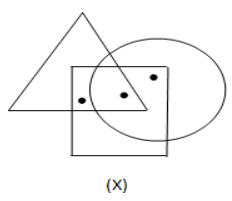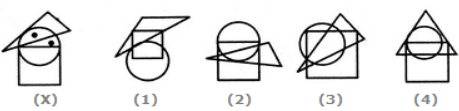# Dot Situation

## DOT SITUATION

The problem on dot situation involve a cluster of three or more geometrical figures-usually triangle, square, rectangle or/and circle one or more dots placed at any point inside the cluster. This cluster is followed by a set of four alternative figures each composed of a cluster of same type of figures. Now, for each dot we have to observe the region in which it is enclosed, i.e.to which of the geometrical figures this region is common, then, we look for such a region in the four alternatives. Once we have found it, we repeat the procedure for other dots if any. The alternative figure which contains all such regions is the answer.Ex. Select the figure which satisfies the same conditions of placement of the dots as in Figure-X.1. 1
2. 2
3. 3
4. 4

Solution: In figure (X), one of the dots lies in the region common to the circle and the triangle only and the other dot lies in the region common to the circle and the square only. In each of the figures (1), (3) and (4), there is no region common to the circle and the triangle only. Only figure (2) consists of both the types of regions. So the answer is (b).